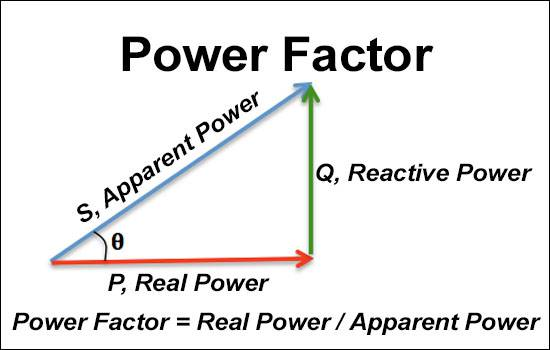﻿ Power Factor

# Power Factor Calculator

Power factor is the ratio of real power absorbed by the load to the apparent power flowing in the circuit and is represented as a number in the range of -1 to 1.

Output values are rounded to the second decimal place. Angle is in degrees.

Power Factor Equation

cosθ = P/S

θ = arctan(Q/P)

Q = √(P-S)

P = Real Power(W)、S = Apparent Power(VA)、Q = Reactive Power(var)、cosθ = Power Factor、θ = Phase AngleCC BY-SA 4.0 Wikieditor4321.

### Input

Real(P):

Apparent(S):

Reactive(Q):

Leave one field blank.